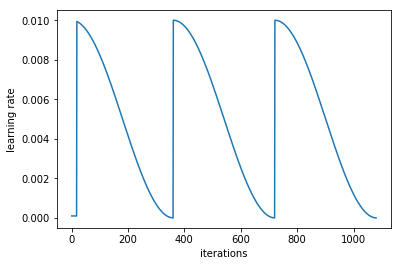# What is an "iteration" in a learning rate finder?

I want to confirm my understanding of the learning rate finder discussed in lessons 1 and 2.

Is each iteration a mini-batch?
I think i heard that with cycle restart the restarts are done on each epoch. Is that correct?

And as a more general question when performing SGD when are weights updated? After each individual sample? After each mini-batch? After each Epoch?

Hi,

That is also my understanding.

The common definition of `epoch` or `num_iteration = num_examples/batch_size` — so each iteration is indeed a mini-batch (and num_iteration make up one epoch or 1 complete pass through the dataset)

If you e.g ran `learn.fit(1e-2, 3, cycle_len=1)` you would see that there are ~360 iterations (=1 epoch) before the lr plot cycles (goes back to 0.01) [360 ~ (11500 cats+11500 dogs)/64]. In this case, each iteration correspond to a single mini-batch of size 64.However, its easy to get confused once you start experimenting with different `cycle_mult`. E.g., if you set `cycle-multi=2` (whilst keeping `cycle_len=1`), during the second cyle you should see about 720 iterations. This is because we went through the dataset twice.

As for your SGD question, in DL one typically uses mini-batch gradient descent (although colloquially its called SGD). It means, the updates are done after each mini-batch. To wit, If you have 64 image/batch, you average your gradient based on 64 examples at a time and update the parameters.

Finally, the old-school(?) SGD updates gradients after each example (here is a fun implementation if you are interested for more)

6 Likes

Thanks for this explanation!
I was just wondering though why the learning rate is updated over the same network over time? In other words, wouldn’t it be better to run seperate models with seperate learning rates instead of updating the learning rate on the same model over time? The problem with the latter I believe is that it is hard to tell what learning rate is best because the losses are based on the previous losses. So for example if learning rate 10-1 performs well it might just be because the sequence of learning rates performed before brought the loss to a point that was just right for the 10-1 learning rate 's performance to bring it to the minimum loss possible. Could you please explain why it is updated over time instead of having seperate learning rates running in parallel? Thanks!

Hi,